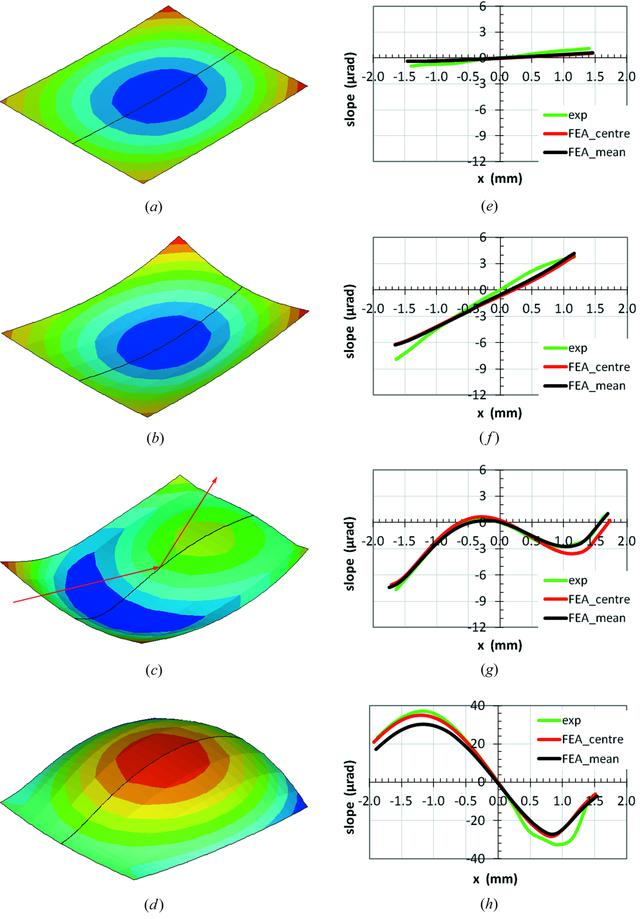disable zoom     view article Figure 9 Left column (a, b, c, d): FEA results for the deformed crystal shapes in the footprint area with the thermal deformation displacement (the scale of the displacement is different for the four cases) in the direction normal to the crystal surface for four heat load conditions on the crystal at beamline ID06: (a, e) P = 19.1 W, Tmax = 85.0 K, (b, f) P = 194.5 W, Tmax = 120.3 K, (c, g) P = 288.9 W, Tmax = 153.6 K, (d, h) P = 385.2 W, Tmax = 205.7 K. The primary slits opening is 2 mm × 1 mm (H × V). The red lines with arrows show the X-ray beam incident and reflecting directions. Right column (e, f, g, h): comparison of calculated and measured thermal slope distributions on the crystal surface in the meridional direction (red line: along the central axis; black line: averaged over the transverse footprint width) for the four heat load conditions. The same scales for the slope distribution are used in (e), (f) and (g); a much larger vertical scale is used for case (h).JOURNAL OFSYNCHROTRONRADIATION
ISSN: 1600-5775
Volume 20| Part 4| May 2013| Pages 567-580